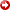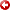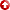[First Hit]HOLBROOK, PHIL, ForceBalancedPetrophysics

Abstract: Pore Pressure Prediction and Detection in Deep Water

General forcebalancedstress/strain physics in the Earth

Pore pressure (Pp) is the fluid load-bearing element in the Earth's subsurface. Deep water settings are particularly amenable to a rigorous forcebalancedapproach for pore pressure detection and prediction. The remaining load borne by a fluid filled rock in the subsurface is the average effective stress (save). The Effective Stress Theorem is the forcebalancedphysical-mathematical expression for porous granular solids that compose the Earth's sedimentary crust. In this rigorous physical expression; the fluid scalar pore pressure (Pp) is the difference between the two solid element scalars average confining load (Save) and average effective stress (save) that is (Pp = Save - save ).

By coincidence, the Terzaghi (1923) mixed scalar-vector relationship (Pp = Sv - sv) works in most Normal Fault Regime basin deep water settings. Terzaghi found empirically that in shallow marine sediments the scalar pore pressure (Pp) was related to the axial load vector, overburden (Sv).

Forces arebalancedin deep water settings, as they are everywhere else in the subsurface. The entire load is both generated and borne by the Earth's matter. The Earth solid-fluid mechanical system is described using a closed form forcebalancedformulation that is an extension of Newtonian physics (Holbrook, 1998). Fluids are the pore pressure transmission system; and solids are the pore fluid pressure regulation and measurement system.

The loading-limb stress/strain relationships are global in nature dependent principally upon mineralogic composition (Holbrook, 1995a). Minerals are the discrete solid load bearing elements of the Earth. Each mineral has a stress/strain coefficient that has been measured in situ under Effective Stress Theorem force balance boundary conditions. These in situ static equilibrium mineralogic: coefficients are related to the average bond strength of that mineral's crystalline lattice. The reversible thermal and elastic stress/strain properties of minerals were measured in laboratories decades ago (Carmichael, 1982).

Solidity (1.0 - f ) is a direct scalar measure of volumetric in situ strain for porous granular solids. Rocks pass into a purely elastic stress/strain regime at the zero porosity limit. During natural loading through burial compaction, sedimentary grains are brought closer together and contact area between grains is increased. The solid element load is borne at these grain contacts and through the mineral lattice to the neighboring grains. Under increasing loads elastic energy is accumulated in the mineral lattice in proportion to strain. Elastic strain is a miniscule fraction of total in situ strain that is dominantly plastic.

Also during natural loading, grain contact area is increased irreversibly following a plastic stress/strain relationship. The limit of plastic compaction is when all fluid filled porosity is.gone and the rock is totally solid. Solidity (1.0 - porosity) is an absolute measure of volumetric in situ strain. Holbrook (1998) provides a physical explanation of the linked forcebalancedstress/strain relationships in the Earth.

Deep Water in situ Petrophysical => Newtonian ForceBalancedmethod to calculate Pore Pressure

Figure 1 shows three flowpaths to calculate water filled porosity from different petrophysical sensors. One minus porosity is absolute in situ strain. Each flowpath depends upon using the appropriate in situ density, conductivity, and elastic coefficients of the minerals and fluid that compose a sedimentary rock. Non-clay mineral coefficients are essentially constant at normal geothermal gradients.The coefficients for clay minerals and Sodium Chloride brines vary with prevailing PV/T and Salinity conditions. The grain density of average sedimentary clay minerals increases gradually with the regional geothermal gradient.

The resistivity and sonic sensor flowpaths contain non-linear pore volume and shape coefficients that must be taken into account in order to calculate porosity. If these sensor => porosity transforms are executed properly and there is no significant borehole wall damage, all three sensor paths should indicate the same in situ True Rock Porosity for water filed rocks. True Rock Porosity is central to many reservoir petrophysical considerations and is the central force balance => strain consideration in figure 1.

Below the True Rock Porosity midpoint on figure 1 is the Newtonian mathematically closed formulation relating force balance to strain in Normal Fault Regime ~ to biaxial basins. The load elements of the Newtonian closed formulation are on the left side of the individually forcebalancedequations. On the left, confining loads are denoted with an (S). Forcebalancedcorresponding effective stress loads are denoted with a (a). Both S and s are subscripted vectors. The "v" subscripts denote vertical gravitational loads and "h" subscripts denote the two corresponding orthogonal horizontal vectorial loads. Average confining load (Save) and average effective stress (save ) are completely three axis (xyz) confined forcebalancedscalars.

The Effective Stress Theorem is the fifth equation in the Newtonian forcebalancedclosed formulation. The scalar Pore pressure (Pp ) is calculated as the difference between the two load scalars [(Save) - (save)]. Fracture propagation pressure (PF = Pp + sh =Sh ) is thereafter calculated in the sixth equation using pore pressure (Pp) calculated using the Effective Stress theorem. All the load terms (S, sh and Pp) to the left of the (=) sips denoted by the sloping dashed line on figure 1 are a Newtonian closed form force balance! The Effective Stress theorem is the missing element in the "Terzaghi" relationship that makes it work.

All the earth strain terms are on the right side of the (=) signs denoted by the sloping dashed line on figure 1. Absolute volumetric in situ strain (1.0 - f ) is in each of the individually forcebalancedstress/strain equations. The descending arrow on figure 1 indicates the algebraic linkage of these equations to petrophysically measurable strain. The remaining strain terms (r, smax and a ) are mineral and fluid coefficients that are compositionally linked to each other. The equal (=) signs denoted by the sloping dashed line on figure 1 mathematically relate forcebalancedloads to absolute in situ strain in the earth! Force balance <=>measurable strain linkage is unique to this Newtonian formulation and leads to simplicity and accuracy of calibration, prediction and detection of pore pressure.

Deep Water basin Geologic correspondences that simplify ForceBalancedPore Pressure Prediction

Seafloor water temperatures are near 4.0 degrees Celsius for water depths below 2000 feet. These waters are essentially normal saline with nearly constant electrical conductivity. Deep water off-shelf depositional environments have been cold water marine for millions of years.These sediments have low thermal al conductivity, have been buried with cold seawater and heated very gradually from below. Water conductivity vs. depth profiles in the deep water off-shelf settings are low, gradual and uniform.

Initial overburden (Sv ) is also highly predictable, as it is mostly seawater. Sediments deposited in cold deep water typically have low carbonate content. Cold water stratigraphic sequences are dominantly a simple binary quartz grainstone claystone mineralogic continuum. A normalized gamma-ray log is a fairly good quartz-clay mineralogic: estimator these settings. Favorable geologic conditions for the petrophysical calculation of overburden (Sv ) and average effective stress (save) are present.

Both Svand save are dependent only on strain, mineral, and fluid coefficients in the Newtonian closed formulation. Effective stress ratio (sh / sv ) is also directly related to (l. - f ) strain. Given these equation redundancies, and the compositionally bound mineral and fluid physical constants, the Newtonian closed formulation is mathematically simple. Given geologic uniformity; pore pressure determination from petrophysical data is further simplified in off-shelf deep water settings.

Figure 2 shows the average formation water conductivity in the Gulf of Mexico 4000 feet below the mudline. Figure 3 is the conductivity increase 8000 feet below the mudline. The water conductivity data was derived from forcebalancedpetrophysical logs at each of the well locations shown on the maps.

The water conductivity contour lines on each map are generally parallel to strike in the Gulf of Mexico basin.The conductivity gradients are more gradual in the colder off-shelf sediments. Decreasing geothermal gradients in the seaward direction are the dominant cause for the mapped patterns. Referring back to figure 1, the  physical quantities needed to calculate pore pressure anywhere along a well depth profile can be calculated from mineral and fluid physical constants and these water conductivity profiles.

Deep Water ForceBalancedPore Pressure/ Fracture Pressure Prediction Method Conclusions

Newtonian closed form forcebalancedpore pressure prediction in deep water settings is mathematically simple and straightforward. The linking coefficients required for in situ strain calibrations are mineral and fluid physical properties. Two variable calibration parameters are needed, initial overburden and a regional water conductivity profile. These are 1) physically representative; 2) Can be determined through measurement; 3) Are continuous between locations that; and 4) Can be estimated through regional mapping.

Fracture pressure is physically linked to pore pressure through strain and force balance in the Newtonian closed formulation. Because of this, leakoff tests serve as additional calibration points for pore pressure when using the Newtonian forcebalancedmethod. The standard deviation of leakoff test measured vs. forcebalancedcalculated fracture pressure is about 0.2 pounds/gallon equivalent mud weight in deep water settings. Leakoff tests are compared to forcebalancedlogs locally to fine-tune the top-of-log initial overburden (Sv) constant and the local water conductivity (Cw) profile. Both types of borehole pressure measurements can be simultaneously calibrated to Newtonian closed form force balance using linked pore pressure/fracture pressure method. The calibration to Newtonian force balance physical approach is the most accurate for both pore pressure and fracture pressure prediction-detection in deep water settings.

References:

Carmichael, R.S., 1982, "Handbook of Physical Properties of Rocks", CRC Press.

Holbrook, P W, 1995a, "The relationship between Porosity, Mineralogy and Effective Stress in Granular Sedimentary Rocks", paper AA in SPWLA 36th Annual Logging Symposium, June 26-29, 1995.

Holbrook, P W, D A Maggiori, and Rodney Hensley, 1995b, "Real-time Pore Pressure and Fracture Pressure Determination in All Sedimentary Lithologies", pp 215 - 222, SPE Formation Evaluation, December 1995.

Holbrook, P W, 1996, "The Use of Petrophysical Data for Well Planning, Drilling Safety and Efficiency it, paper X in SPWLA 37th Annual Logging Symposium, June 16-19, 1996.

Holbrook, P W, 1998, "Physical explanation of the dosed form mineralogic forcebalancedstress/strain relationships in the Earth's sedimentary crust." presented at Overpressures in Petroleum Exploration, the European International Pore Pressure Conference, April 7-8 Pau., France.

Terzaghi, K.Van, 1923, "Die Berchnung der Durchassigkeitziffer des Tones aus dem Verlauf der Hydrodynamischen Spannungscheinungen", Sitzunzsber Akad Wiss.Wein Math Naturwiss, K1.ABTS 2a, pp. 107-122.

Figure 1. Linkage of petrophysical sensor readings through mineralogically sensitive porosity transforms to the Newtonian closed form forcebalancedstress/strain relationship in deep water NFR <is approx. equal to> biaxial basins.

Figure 2. Average increase in formation water conductivity (mhos) 4000 feet below the mudline in the Gulf of Mexico.

Figure 3. Average increase in formation water conductivity (mhos) 8000 feet below the mudline in the Gulf of Mexico.

AAPG Search and Discovery Article #[email protected] International Conference and Exhibition, Birmingham, England